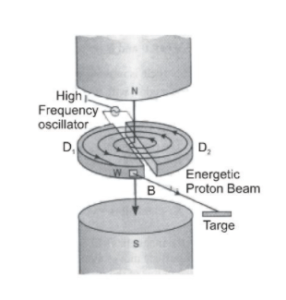Deepak Scored 45->99%ile with Bounce Back Crack Course. You can do it too!

# Construction and Working Principle of Cyclotron Class 12 Physics

We will study here about the Construction and Working Principle of Cyclotron Class 12

## Cyclotron Introduction

A cyclotron is used for accelerating positive ions so that they acquire energy large enough to carry out nuclear reactions.Cyclotron was designed by Lawrence and Livingstone in 1931.

In a cyclotron, the positive ions cross again and again the same alternating (radio frequency) electric field.

And gain the energy each time = q V.

q = charge and $V=p o t^{n}$ . difference in betn dees.

It is achieved by making them to move along the spiral paths under the action of a strong magnetic field.

## Working Principle of Cyclotron

A positive ion can acquire sufficiently large energy with a comparatively smaller alternating potential difference by making them to cross the same electric field again and again by making use of a strong magnetic field.

### Construction of Cyclotron

It consists of two D-shaped hollow semicircular metal chambers $\mathrm{D}_{1}$ and $\mathrm{D}_{2}$, called dees.

The two dees are placed horizontally with a small gap separating them.

The dees are connected to the source of the high-frequency electric field.

The dees are enclosed in a metal box containing a gas at a low pressure of the order of

10–3 mm mercury.

The whole apparatus is placed between the two poles of a strong electromagnet NS as shown in fig. The magnetic field acts perpendicular to the plane of the dees.

The positive ions are produced in the gap between the two dees by the ionization of the gas.

To produce a proton, hydrogen gas is used; while for producing $\alpha$ -particles, helium gas is used. Theory: Consider that a positive ion is produced at the center of the gap at the time, when the dee $D_{1}$ is at a positive potential and the dee $D_{2}$, is at a negative potential. The positive ion will move from dee $D_{1}$ to dee $D_{2}$ The force on the positive ion due to the magnetic field provides the centripetal force to the positive ion and it is deflected along a circular path because the magnetic field is normal to the motion. Let strength of the magnetic field $=\mathrm{B}$ mass of ion $=\mathrm{m}, \quad$ velocity of ion $=\mathrm{v} \quad$ and $\quad$ charge of the positive ion $=\mathrm{q}$ and the radius of the semi-circular path $=\mathrm{r}$

then $\left.\quad \mathrm{Bqv}=\frac{\mathrm{mv}^{2}}{\mathrm{r}} \quad \text { [inside the dee } \mathrm{D}_{2}\right]$

Thus, $r=\frac{m v}{B q}$

After moving along the semi-circular path inside the dee $D_{2},$ the positive ion reaches the gap between the dees.

At this stage, the polarity of the dees just reverses due to alternating "electric field" i.e. dee $D_{1}$, becomes negative and dee $\mathrm{D}_{2}$ becomes positive. The positive ion again gains the energy, as it is attracted by the dee $D_{1}$, After moving along the semi-circular path inside the dee $D_{1}$, the positive ion again reaches the gap and it gains the energy. ( $=\mathrm{q} \mathrm{V}$ ) This process repeats itself because the positive ion spends the same time inside a dee irrespective of its velocity or the radius of the circular path.

The time spent inside a dee to cover semi-circular path,

is $\quad \mathrm{t}=\frac{\text { length of the semi circular path }}{\text { velocity }}=\frac{\pi \mathrm{r}}{\mathrm{v}}$

Or $\mathrm{t}=\frac{\pi \mathrm{m}}{\mathrm{Bq}} \quad\left[\frac{\mathrm{r}}{\mathrm{v}}=\frac{\mathrm{m}}{\mathrm{Bq}}\right]$

As positive ion gains kinetic energy its velocity increases, due to increasing velocity, decrease in time spent inside a dee of positive ions is exactly compensated by the increase in length of the semi circular path (r $\propto$ v).

Due to this condition, the positive ion always crosses the alternating electric field across the gap incorrect phase.